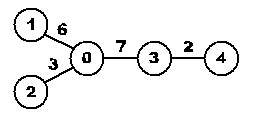## Дата и время

04/06/2023 12:08:04

## Авторизация

 Имя: Пароль:
Зарегистрироваться
Восстановить пароль

#Тренировка 3

##A. Average distance

Ограничения: время – 3s/6s, память – 64MiB Ввод: input.txt или стандартный ввод Вывод: output.txt или стандартный выводПослать решение Blockly Посылки Темы Где Обсудить (0)

Given a tree, calculate the average distance between two vertices in the tree. For example, the average distance between two vertices in the following tree is (d_01\ +\ d_02\ +\ d_03\ +d_04\ +\ d_12\ +\ d_13\ +\ d_14\ +\ d_23\ +\ d_24\ +\ d_34)/10\ =\ (6+3+7+9+9+13+15+10+12+2)/10\ =\ 8.6.Figure 1: The first sample case
Input
On the first line an integer t (1\ ≤\ t\ ≤\ 100): the number of test cases. Then for each test case:
One line with an integer n (2\ ≤\ n\ ≤\ 10000): the number of nodes in the tree. The nodes are numbered from 0 to n 1.
n-1 lines, each with three integers a (0\ ≤\ a\ <\ n), b (0\ ≤\ b\ <\ n) and d (1\ ≤\ d\ ≤\ 1000). There is an edge between the nodes with numbers a and b of length d. The resulting graph will be a tree.
Output
For each testcase:
One line with the average distance between two vertices. This value should have an absolute error of at most 10^{-3}.

Sample Input

1
5
0 1 6
0 2 3
0 3 7
3 4 2


Sample Output

8.600

Source: Benelux Algorithm Programming Contest 2007